Representation of Strong Entity SetsNext: Representation of Weak Up: Reducing E-R Diagrams Previous: Reducing E-R Diagrams

## Representation of Strong Entity Sets

We use a table with one column for each attribute of the set. Each row in the table corresponds to one entity of the entity set. For the entity set account, see the table of figure 2.14.

We can add, delete and modify rows (to reflect changes in the real world).

A row of a table will consist of an n-tuple where n is the number of attributes.

Actually, the table contains a subset of the set of all possible rows. We refer to the set of all possible rows as the cartesian product of the sets of all attribute values.

We may denote this as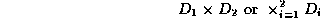for the account table, where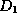and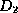denote the set of all account numbers and all account balances, respectively.

In general, for a table of n columns, we may denote the cartesian product of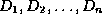by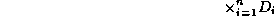Page created and maintained by Osmar R. Zaï ane
Last Update: Sun Sep 10 17:02:35 PDT 1995